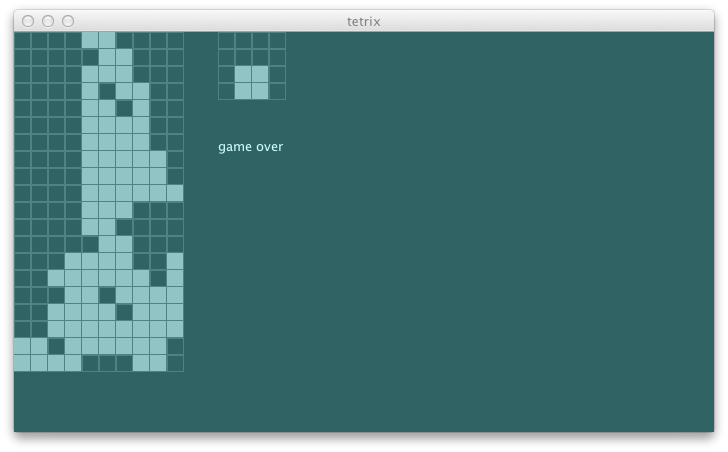### buggy penalty

Second suspicion was that there was something wrong with the penalty calculation. Let’s add more test:

``````    """penalize having gaps between the columns"""          ! utility4^
...
def utility4 = {
val s = newState(Seq(
(0, 0), (0, 1), (0, 2), (0, 3), (0, 4), (0, 5), (0, 6))
map { Block(_, TKind) }, (10, 20), TKind :: TKind :: Nil)
agent.utility(s) must_== -36.0
} and {
val s = newState(Seq((1, 0), (1, 1), (2, 1), (2, 2))
map { Block(_, ZKind) }, (10, 20), TKind :: TKind :: Nil)
agent.utility(s) must_== -13.0
}
``````

And it fails.

``````[error] x penalize having gaps between the columns
[error]    '-4.0' is not equal to '-13.0' (AgentSpec.scala:35)
``````

Before we go into the REPL, we can save some typing by adding the following to `build.sbt`:

``````initialCommands in console := """import com.eed3si9n.tetrix._
|import Stage._""".stripMargin,
``````

After reloading, type in `console` from sbt shell and open the REPL:

``````[info]
import com.eed3si9n.tetrix._
import Stage._
Welcome to Scala version 2.10.2 (Java HotSpot(TM) 64-Bit Server VM, Java 1.6.0_51).
Type in expressions to have them evaluated.
Type :help for more information.

scala> move // hit tab key
moveLeft    moveRight
``````

Yes, the tab key completion works in the REPL.

``````scala>     val s = newState(Seq((1, 0), (1, 1), (2, 1), (2, 2))
map { Block(_, ZKind) }, (10, 20), TKind :: TKind :: Nil)
s: com.eed3si9n.tetrix.GameState = GameState(List(Block((1,0),ZKind), Block((1,1),ZKind),
Block((2,1),ZKind), Block((2,2),ZKind), Block((4,18),TKind), Block((5,18),TKind),
Block((6,18),TKind), Block((5,19),TKind)),(10,20),
Piece((5.0,18.0),TKind,List((-1.0,0.0), (0.0,0.0), (1.0,0.0), (0.0,1.0))),
Piece((2.0,1.0),TKind,List((-1.0,0.0), (0.0,0.0), (1.0,0.0), (0.0,1.0))),List(),ActiveStatus,0)

scala>     val heights = s.unload(s.currentPiece).blocks map {
_.pos} groupBy {_._1} map { case (k, v) => (k, v.map({_._2}).max) }
heights: scala.collection.immutable.Map[Int,Int] = Map(1 -> 1, 2 -> 2)

scala>     val gaps = (0 to s.gridSize._1 - 2).toSeq map { x =>
heights.getOrElse(x, 0) - heights.getOrElse(x + 1, 0) } filter {_ > 1}
gaps: scala.collection.immutable.IndexedSeq[Int] = Vector(2)
``````

Off by one error! Did you catch this? The lowest coordinate is `0`, yet I am returning `0` as the default.

Also I am throwing out the negative numbers. The correct `gap` should be:

``````scala>     val gaps = (0 to s.gridSize._1 - 2).toSeq map { x =>
heights.getOrElse(x, -1) - heights.getOrElse(x + 1, -1) } filter {math.abs(_) > 1}
gaps: scala.collection.immutable.IndexedSeq[Int] = Vector(-2, 3)
``````

I didn’t catch this because my hand calculated value of -36.0 was also incorrect, which should have been -49.0. Now all tests pass:

``````[info] + penalize having gaps between the columns
``````

It feels more logical now, but the penalty isn’t nudging the game to actual scoring or creating the right set up. First, I want to separate the reward component and penalty component of the utility function so it’s easier to test. Second, instead of the gaps, let’s try penalizing the heights in general.

``````                                                                              s2"""
Penalty function should
penalize having blocks stacked up high                                    \$penalty1
"""
...
def penalty1 = {
val s = newState(Seq(
(0, 0), (0, 1), (0, 2), (0, 3), (0, 4), (0, 5), (0, 6))
map { Block(_, TKind) }, (10, 20), TKind :: TKind :: Nil)
agent.penalty(s) must_== 49.0
} and {
val s = newState(Seq((1, 0))
map { Block(_, ZKind) }, (10, 20), TKind :: TKind :: Nil)
agent.penalty(s) must_== 1.0
}
``````

Here’s the new `penalty`:

``````  def penalty(s: GameState): Double = {
val heights = s.unload(s.currentPiece).blocks map {
_.pos} groupBy {_._1} map { case (k, v) => v.map({_._2 + 1}).max }
heights map { x => x * x } sum
}
``````

Another thing I want to tweak is the balance between the reward and penalty. Currently the incentive of deleting the line is too little compared to avoiding the penalty.

``````  def utility(state: GameState): Double =
if (state.status == GameOver) minUtility
else reward(state) - penalty(state) / 10.0
``````

This at least made the agent delete a line in the following game: